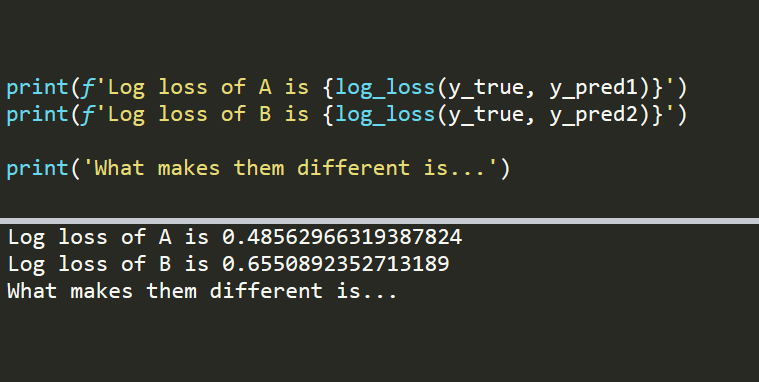# Why We Care About the Log LossThe most common metric used in Kaggle competitions. In this post, we will see what makes the log loss the number one choice. Before we start on the examples, let’s briefly explain what the log loss is.

The most critical part of a machine learning pipeline is performance evaluation. A robust and thorough evaluation process is required to understand the performance and shortcomings of a model.

When it comes to a classification task, log loss is one of the most commonly used metrics. It is also known as the cross-entropy loss. If you follow or join Kaggle competitions, you will see that log loss is the predominant choice of evaluation metrics.

In this post, we will see what makes the log loss the number one choice. Before we start on the examples, let’s briefly explain what the log loss is.

Log loss (i.e. cross-entropy loss) evaluates the performance by comparing the actual class labels and the predicted probabilities. The comparison is quantified using cross-entropy.

Cross-entropy quantifies the comparison of two probability distributions. In supervised learning tasks, we have a target variable that we are trying to predict. The actual distribution of the target variable and our predictions are compared using the cross-entropy. The result is the cross-entropy loss, also known as the log loss.

When calculating the log loss, we take the negative of the natural log of predicted probabilities. The more certain we are at the prediction, the lower the log loss (assuming the prediction is correct).

For instance, -log(0.9) is equal to 0.10536 and -log(0.8) is equal to 0.22314. Thus, being 90% sure results in a lower log loss than being 80% sure.

I explained the concepts of entropy, cross-entropy, and log loss in detail in a separate post if you’d like to read further. This post is more like a practical guide to show what makes the log loss so important.

In a classification task, models usually output a probability value for each class. Then the class with the highest probability is assigned as the predicted class. The traditional metrics like classification accuracy, precision, and recall evaluates the performance by comparing the predicted class and actual class.

Consider the following case.

``````import numpy as np

y_true = np.array([1,0,0,1,1])``````

## 15 Machine Learning and Data Science Project Ideas with Datasets

Learning is a new fun in the field of Machine Learning and Data Science. In this article, we’ll be discussing 15 machine learning and data science projects.

## Most popular Data Science and Machine Learning courses — July 2020

Most popular Data Science and Machine Learning courses — August 2020. This list was last updated in August 2020 — and will be updated regularly so as to keep it relevant

## Artificial Intelligence vs Machine Learning vs Data Science

Artificial Intelligence, Machine Learning, and Data Science are amongst a few terms that have become extremely popular amongst professionals in almost all the fields.

## Predict Diabetes with Machine Learning | Data Science | Machine Learning | Python

About one in seven adults in the United States have DiabetesIn this article, I will show you how you can use machine learning to Predict Diabetes using Pyth

## AI(Artificial Intelligence): The Business Benefits of Machine Learning

Enroll now at CETPA, the best Institute in India for Artificial Intelligence Online Training Course and Certification for students & working professionals & avail 50% instant discount.